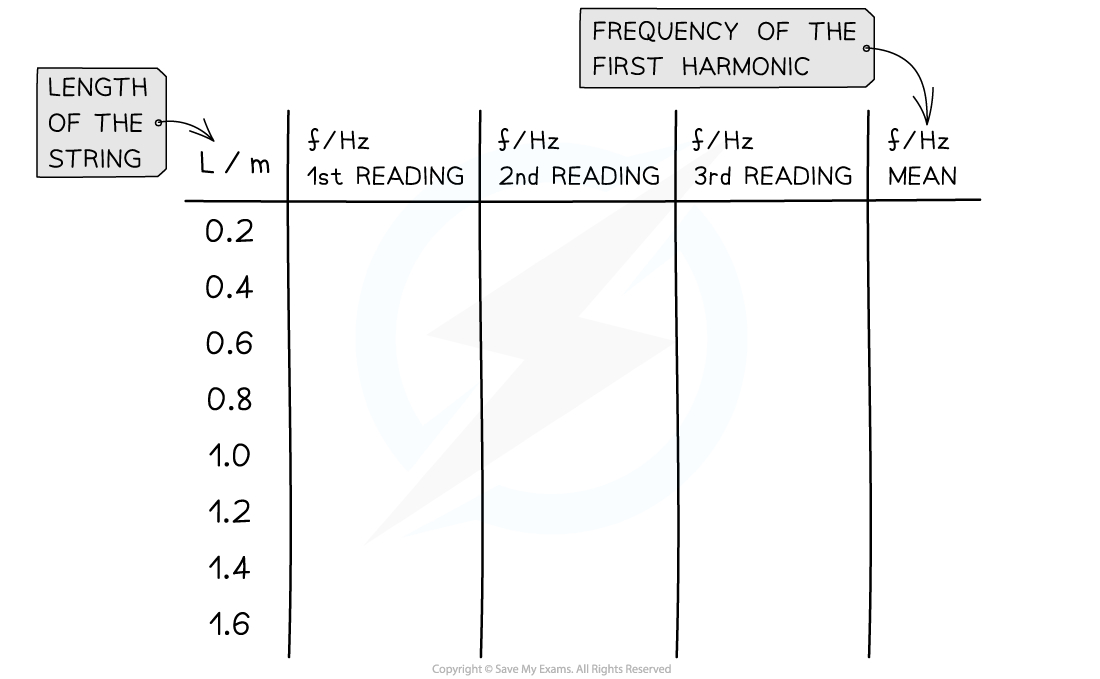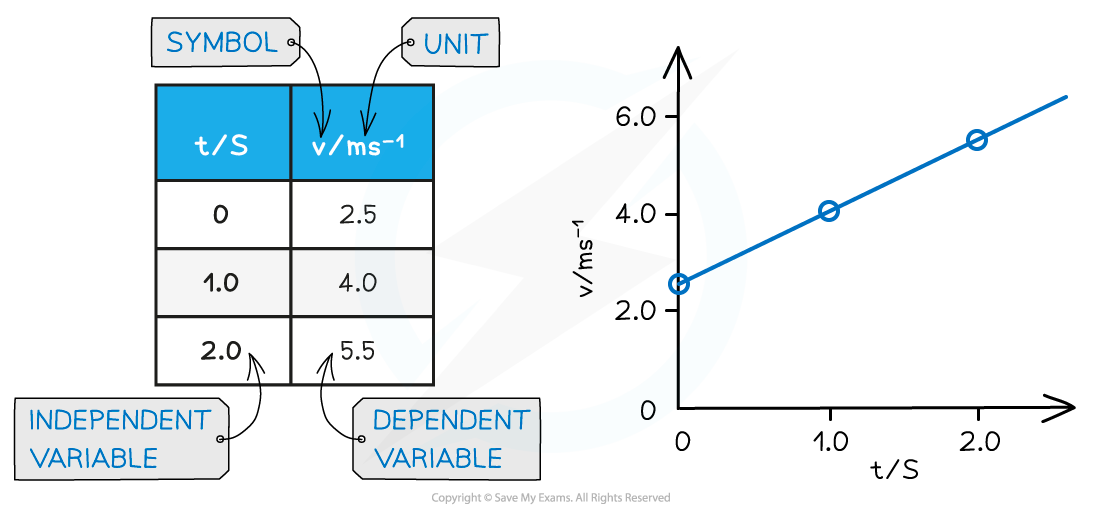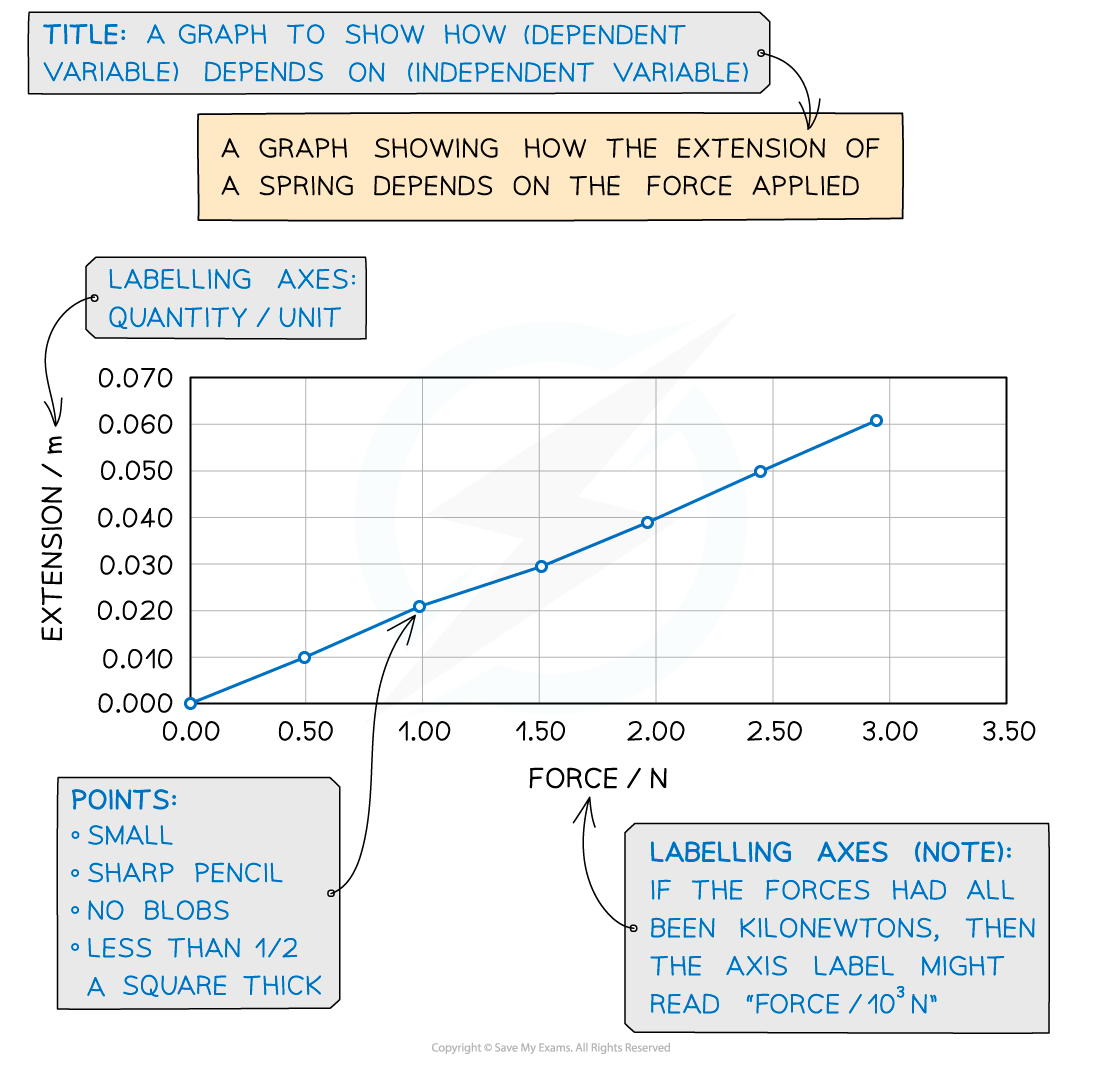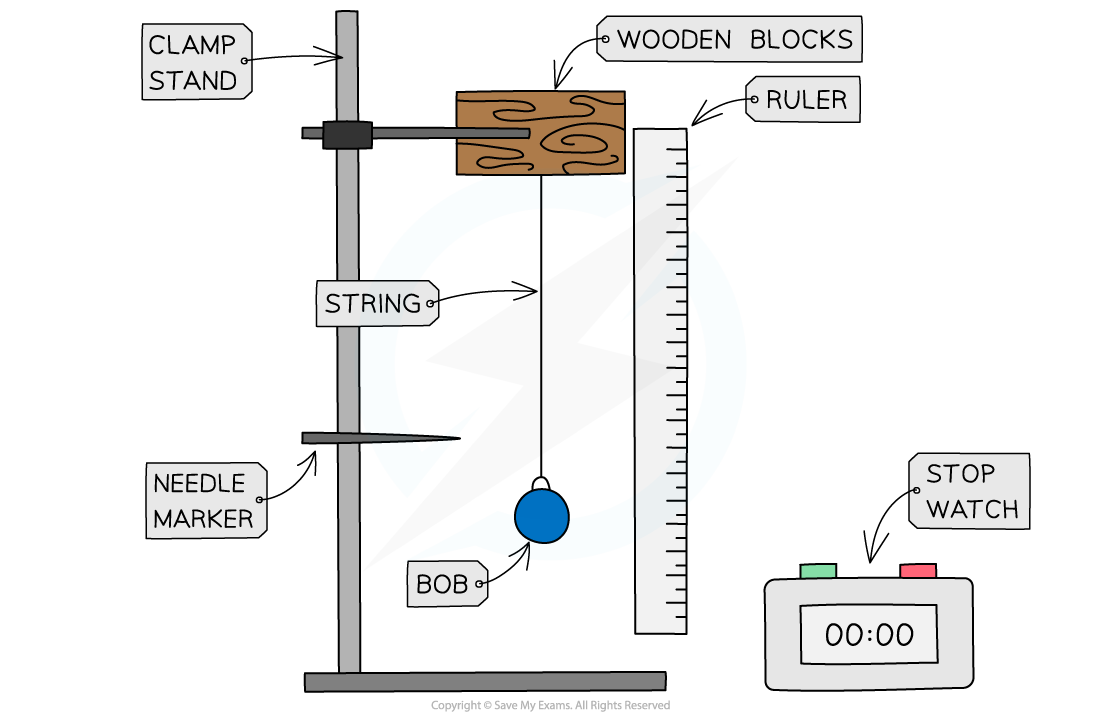# Edexcel A Level Physics:复习笔记1.5 Scientific Communication

### Scientific Communication

• Scientific communication of the results of an experiment are extremely important
• The ideas must be communicated in an appropriate way using appropriate terminology
• This involves using terms such as accuracy, validity and stating the sources of random and systematic errors
• Scientists have to design an experiment to answer a question or investigate something
• These will involve dependent and independent variables
• An independent variable is what is changed
• A dependent variable is what is measured
• The control variables are what do not change
• For example, in an investigation of the variation of potential difference and current across a light bulb
• The independent variable is the potential difference
• The dependent variable is the current
• The control variable would be the temperature of the apparatus
• Data must always be presented in a scientific way
• This may include:
• Tables
• Graphs
• Diagrams

#### Presenting Data in Tables

• When taking readings, a sensible range should be taken, and the values should all be stated to an appropriate number of significant figures or decimal places
• This is usually the same number as the resolution of the measuring instrument

• The columns in any table should have both a quantity and a unit in their heading
• When labelling columns, the names of the quantities should be separated from their unit by a forward slash ( / )

• For data displayed in a table:
• The first column should contain the independent variable
• The second column should contain the dependent variable
• If repeat readings of the dependent variable are required, these should be included with a column for the mean value at the end
• Any columns required for processing data e.g. calculations should come after thisConventions for presenting data in a table. The length is the independent variable and the frequency is the dependent variable

• In summary, when presenting tables the following must be included:
• Clear headings, or symbols, for columns
• Relevant units for measurements
• Readings listed to the same number of significant figuresAn example of a correctly labelled table with corresponding graph

#### Presenting Data on a Graph

• All readings, including suspected anomalous results, should be plotted on a graph so that they can be easily identified
• When taking repeat readings, it is the mean value that is plotted
• The way data is presented on a graph depends on what type of data it is

Discrete data

• Only certain values can be taken, normally a whole number e.g. number of students
• This should be displayed on a scatter graph or bar chart

Continuous data

• Can take any value on a scale e.g. voltage in a circuit
• This should be displayed on a line or scatter graph

Categorical data

• Values that can be sorted into categories e.g. types of material
• This should be displayed on a pie or bar chart

Ordered data

• Data that can be put in ordered categories e.g. low, medium, high
• This should be displayed on a bar chart

• In summary, when presenting graphs the following must be included:
• An explanatory title
• Clearly labeled axes
• Relevant units for measurements
• Well plotted points
• A smooth line or curve of best fitAn example of a correctly labelled and plotted graph

#### Presenting Diagrams

• When presenting diagrams, such as apparatus set-up, all the relevant parts must be clearly labelledAn appropriately labeled diagram of the set-up of an investigation into simple harmonic motion